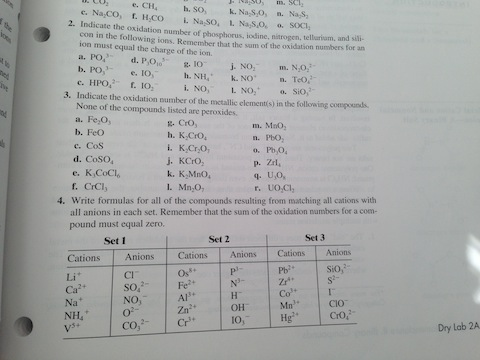# Indicate the oxidation number of phosphorus, iodine, nitrogen, tellurium, and sillicon in the following ions.

Discipline: Chemistry

Paper Format: APA

Pages: 1 Words: 275

Question

Indicate the oxidation number of phosphorus, iodine, nitrogen, tellurium, and sillicon in the following ions. Remember that the sum of the oxidation numbers for an ion must equal the charge of the ion. PO43- PO33- HPO42- P3O103- IO3- IO2- IO- NH4+ NO2+ NO2- NO- NO3+ N2O22- TeO42- SiO22- Indicate the oxidation number of the metallic element(s) in the following compounds. None of the compounds listed are peroxides. Fe2O3 FeO CoS CoSO4 K3CoCl6 CrCl3 CeO3 K2CrO4 K2Cr2O7 KCrO2 K2MnO4 Mn2O7 MnO2 PbO2 Pb3O4 Zrl4 U3O2 UO2Cl2 Write formulas for all of the compounds resulting from matching all cations with all anions in each set. Remember that the sum of the oxidation numbers for a compound must equal zero.2] a.P=8-3=5

b.P=6-3=3

c.P=8-2-1=5

d. P=+5

e.I=6-1=5

f.I=4-1=3

g. I=2-1=1

h.N=-3

i.N=4-1=3

j.N=4-1=3

k.N=1

l.N=+3

m.N=+1

n.Te=+6

0.Si=+4

3]a.Fe=+3

b.Fe =+2

c. Co=+2

d.Co=+2

e.Co=+3

f.Cr=+3

g.Cr=+6

h.Cr=+6

i. Cr=+6

j.Cr=+3

k.Mn=+6

l.Mn=+7

m.Mn=+4

n.Pb=+4

o. Pb=+4

p.Zr=+4

r.U=+6

4. a]LiCl

b]CaSo4

c]NaNo3

d][NH4]2O

e] V2{CO3}5

f]Os3P8

g]Fe3N2

h]AlH3

i]ZnOH2

j]Cr[Io3]3

k]PbSiO3

l]ZrS2

m}CoI3

n]MnClO3

o]HgCro4